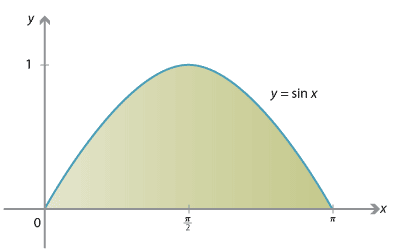## Content

### Integrating trigonometric functions

Since integration is the reverse of differentiation, we have immediately that

$\int \cos x\, dx = \sin x + C \qquad\text{and}\qquad \int \sin x\, dx = -\cos x + C.$

Thus, for example, we can find the area under the sine curve between $$x=0$$ and $$x=\pi$$, as shown on the following graph.

$\text{Area} = \int_0^\pi \sin x\, dx = \bigl[-\cos x\bigr]_0^\pi = 2.$Detailed description

More generally, since

$\dfrac{d}{dx} \sin(ax+b) = a\,\cos(ax+b) \quad\text{and}\quad \dfrac{d}{dx} \cos(ax+b) = -a\,\sin(ax+b),$

we obtain, for $$a\neq 0$$,

$\int \cos (ax+b)\, dx = \dfrac{1}{a}\sin (ax+b) + C \quad\text{and}\quad \int \sin (ax+b)\, dx = -\dfrac{1}{a}\cos (ax+b) + C.$

From $$\dfrac{d}{dx} (\tan x) = \sec^2x$$, we obtain

$\int \sec^2 x\, dx = \tan x + C.$

#### Example

Find

1. $$\displaystyle \int_0^{\frac{\pi}{2}} \bigl(1+\cos 2x\bigr)\, dx$$
2. $$\displaystyle \int_0^{\frac{\pi}{6}} \bigl(\sin 3x+\sec^2 2x\bigr)\, dx$$.

#### Solution

1. $$\displaystyle \int_0^{\frac{\pi}{2}} \bigl(1+\cos 2x\bigr)\, dx = \Bigl[x+\dfrac{1}{2}\sin 2x\Bigr]_0^{\frac{\pi}{2}} = \dfrac{\pi}{2}$$
2. $$\displaystyle \int_0^{\frac{\pi}{6}} \bigl(\sin 3x+\sec^2 2x\bigr)\, dx = \Bigl[-\dfrac{1}{3}\cos 3x + \dfrac{1}{2}\tan 2x \Bigr]_0^{\frac{\pi}{6}} = \dfrac{1}{3} + \dfrac{\sqrt{3}}{2}$$.

Exercise 10

1. Find $$\displaystyle \int_{\frac{\pi}{6}}^{\frac{\pi}{3}} (\sin 2x + \cos 3x)\, dx$$.
2. Differentiate $$x\,\sin x$$, and hence find $$\displaystyle \int_0^\frac{\pi}{2} x\,\cos x\, dx$$.
3. Use the identity $$1+\tan^2x = \sec^2 x$$ to find $$\displaystyle \int \tan^2 x\, dx$$.

#### Special integrals

The two integrals

$\int \cos^2 \theta\, d\theta \qquad\text{and}\qquad \int \sin^2 \theta\, d\theta$

require some special attention. They are handled in similar ways.

To proceed, we make use of two trigonometric identities (a double-angle formula and the Pythagorean identity):

$\cos^2 \theta - \sin^2\theta = \cos 2\theta \qquad\text{and}\qquad \cos^2\theta + \sin^2\theta = 1.$

Adding these two identities, we have $$2\cos^2\theta = 1+ \cos2\theta$$, and so we can replace $$\cos^2\theta$$ in the integral with $$\dfrac{1}{2}(1+\cos 2\theta)$$. Thus

$\int \cos^2 \theta\, d\theta = \int \dfrac{1}{2}(1+\cos 2\theta)\, d\theta = \dfrac{1}{2}\Bigl(\theta + \dfrac{1}{2}\sin 2\theta\Bigr) + C.$

Exercise 11

Find $$\displaystyle \int \sin^2 \theta\, d\theta$$. (Follow the method used for $$\cos^2\theta$$, but subtract the two identities rather than adding them.)

Next page - Content - Simple harmonic motion